# Absorption models

##### 2021-08-10
using Random
using Pumas
using PlottingUtilities
using PumasPlots
using CairoMakie

Random.seed!(1234)  # Set random seed for reproducibility

MersenneTwister(1234)


## Introduction

Absorption modeling is an integral part of pharmacokinetic modeling for drugs delivered by any route of administration other than intravenous (IV) (e.g. oral, subcutaneous, intramuscular, transdermal, nasal, etc.). In the simplest case, drugs administered orally may undergo first-order absorption, whereby the absorption from the depot compartment (i.e. gut) to the central compartment (i.e. plasma) is a first-order process. However, many more complex situations arise in practice, some of which will be covered in this tutorial.

In this tutorial, we will cover the following absorption models:

• First-order

• Zero-order

• Parallel zero-order and first-order

• Two parallel first-order processes

• Weibull-type absorption

• Absorption through a sequence of transit compartments (Erlang absorption)

## Generating the Population

We begin by simulating a population of 10 subjects to use for illustration purposes in this tutorial. For simplicity, we will use a 1-compartment distribution system with additional absorption compartment(s) as required. Note that in these examples we are simulating without residual error (it could be added, but isn't necessary here). Hence, we use the @observed block that is designed to capture post dynamics outputs.

dose = DosageRegimen(100, time = 0)

choose_covariates() = (wt = rand(55:80), dose = 100)

subj_with_covariates = map(1:10) do i
Subject(id = i,
events = dose,
covariates = choose_covariates(),
observations = (conc = nothing,))
end

Population
Subjects: 10
Covariates: wt, dose
Observations: conc


For a more in-depth discussion of simulating data with Pumas, please see the tutorial generating and simulation populations.

## First-Order Absorption

Here is the Pumas code for a 1-compartment model with first-order absorption, diagonal random-effects structure, and allometric scaling on clearance and volume:

foabs = @model begin

@param begin
tvcl ∈ RealDomain(lower=0)
tvvc ∈ RealDomain(lower=0)
tvka ∈ RealDomain(lower=0)
Ω    ∈ PDiagDomain(3)
end

@random begin
η ~ MvNormal(Ω)
end

@covariates wt

@pre begin
CL = tvcl*(wt/70)^0.75*exp(η)
Vc = tvvc*(wt/70)*exp(η)
Ka = tvka*exp(η)
end

@dynamics begin
Depot'   = -Ka*Depot
Central' =  Ka*Depot - (CL/Vc)*Central
end

@observed begin
conc = @. Central/Vc
end
end

PumasModel
Parameters: tvcl, tvvc, tvka, Ω
Random effects: η
Covariates: wt
Dynamical variables: Depot, Central
Derived:
Observed: conc


Note that we have written the model using differential equations, but we could equally have specified an "analytical" (closed-form) solution using this @dynamics block instead (see documentation on analytical solutions):

@dynamics Depots1Central1


Below are a set of parameter values for this model:

param = (
tvcl = 5, tvvc = 20, tvka = 1,
Ω = Diagonal([0.04, 0.04, 0.04]))

(tvcl = 5, tvvc = 20, tvka = 1, Ω = [0.04 0.0 0.0; 0.0 0.04 0.0; 0.0 0.0 0.
04])


We can now use the data and model with parameters to simulate a first-order absorption profile:

sims = simobs(foabs, subj_with_covariates, param, obstimes = 0:.1:24)


And now, we plot the results:

sim_plot(foabs,
sims, observations =[:conc],
figure = (fontsize = 18, ),
axis = (xlabel = "Time (hr)",
ylabel = "Predicted Concentration (ng/mL)",
title = "First-order absorption"))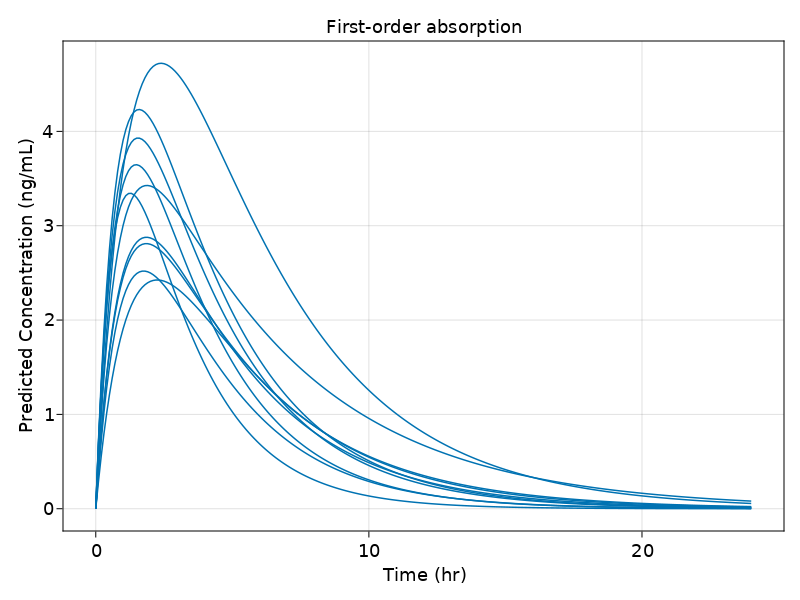## Zero-Order Absorption

Zero-order absorption is less common than first-order absorption. It is essentially like an IV infusion, but where the duration of the infusion is an estimated parameter rather than a known quantity. Here is the Pumas code for the same 1-compartment model but with zero-order absorption:

zoabs = @model begin
@param begin
tvcl  ∈ RealDomain(lower=0)
tvvc  ∈ RealDomain(lower=0)
tvdur ∈ RealDomain(lower=0)
Ω     ∈ PDiagDomain(3)
end

@random begin
η ~ MvNormal(Ω)
end

@covariates wt

@pre begin
CL = tvcl*(wt/70)^0.75*exp(η)
Vc = tvvc*(wt/70)*exp(η)
end

@dosecontrol begin
duration = (Central = tvdur*exp(η),)
end

@dynamics begin
Central' =  - (CL/Vc)*Central
end

@observed begin
conc = @. Central/Vc
end

end

PumasModel
Parameters: tvcl, tvvc, tvdur, Ω
Random effects: η
Covariates: wt
Dynamical variables: Central
Derived:
Observed: conc


Notice that there is no depot compartment, and that instead the absorption takes place in the @dosecontrol block by specifying a duration for the zero-order process. In order for this to work, we need to set the rate data item in DosageRegimen to the value -2; this is a clue to the Pumas engine that the rate should be derived from the duration and amt.

Below are a set of parameter values for this model:

param = (
tvcl = 0.792, tvvc = 13.7, tvdur = 5.0,
Ω = Diagonal([0.04, 0.04, 0.04]))

(tvcl = 0.792, tvvc = 13.7, tvdur = 5.0, Ω = [0.04 0.0 0.0; 0.0 0.04 0.0; 0
.0 0.0 0.04])


We can now use the data and model with parameters to simulate a zero-order absorption profile:

dose = DosageRegimen(100, time = 0, rate = -2)  # Note: rate must be -2 for duration modeling

subj_with_covariates = map(1:10) do i
Subject(id = i,
events = dose,
covariates = choose_covariates(),
observations = (conc = nothing,))
end

sims = simobs(zoabs, subj_with_covariates, param, obstimes = 0:0.1:48)


And now, we plot the results:

sim_plot(zoabs,
sims, observations =[:conc],
figure = (fontsize = 18, ),
axis = (xlabel = "Time (hr)",
ylabel = "Predicted Concentration (ng/mL)",
title = "Zero-order absorption"))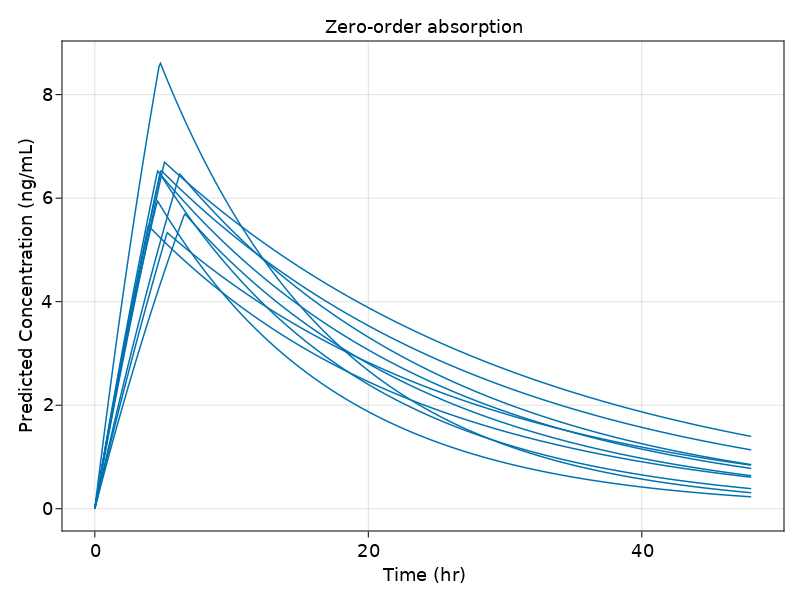## Parallel Zero-Order and First-Order Absorption

This is a more complex absorption model, with both a zero-order process and a first-order process operating simultaneously. Essentially, the total dose is split into two parts, one of which undergoes first-order absorption and the other zero-order absorption (how this splitting is typically accomplished from a technical perspective is to treat each actual dose as two virtual doses with different bioavailable fractions, as in the example below). Furthermore, we will assume that the first-order process is subject to a lag (i.e., the absorption begins after a certain time delay, which is a model parameter).

zofo_paral_abs = @model begin

@param begin
tvcl  ∈ RealDomain(lower=0)
tvvc  ∈ RealDomain(lower=0)
tvka  ∈ RealDomain(lower=0)
tvdur ∈ RealDomain(lower=0)
tvbio ∈ RealDomain(lower=0)
tvlag ∈ RealDomain(lower=0)
Ω     ∈ PDiagDomain(2)
end

@random begin
η ~ MvNormal(Ω)
end

@covariates wt

@pre begin
CL = tvcl*(wt/70)^0.75*exp(η)
Vc = tvvc*(wt/70)*exp(η)
Ka = tvka
end

@dosecontrol begin
duration = (Central = tvdur,)
bioav    = (Depot = tvbio, Central = 1 - tvbio)
lags     = (Depot = tvlag,)
end

@dynamics begin
Depot'   = -Ka*Depot
Central' =  Ka*Depot - (CL/Vc)*Central
end

@observed begin
conc = @. Central/Vc
end

end

PumasModel
Parameters: tvcl, tvvc, tvka, tvdur, tvbio, tvlag, Ω
Random effects: η
Covariates: wt
Dynamical variables: Depot, Central
Derived:
Observed: conc


Below are a set of parameter values for this model:

param = (
tvcl = 5, tvvc = 50, tvka = 1.2, tvdur = 2, tvbio = 0.5, tvlag = 1,
Ω = Diagonal([0.04, 0.04]))

(tvcl = 5, tvvc = 50, tvka = 1.2, tvdur = 2, tvbio = 0.5, tvlag = 1, Ω = [0
.04 0.0; 0.0 0.04])


With these parameter values, the first-order process starts 1 hour after administration, while the zero-order process lasts 2 hours. Hence, there is one hour where they overlap.

We can now use the data and model with parameters to simulate a parallel first- and zero-order absorption profile:

dose_zo = DosageRegimen(100, time = 0, cmt = 2, rate = -2, evid = 1)  # Note: rate must be -2 for duration modeling
dose_fo = DosageRegimen(100, time = 0, cmt = 1, rate = 0, evid = 1)
dose    = DosageRegimen(dose_fo, dose_zo)  # Actual dose is made up of 2 virtual doses

subj_with_covariates = map(1:10) do i
Subject(id = i,
events = dose,
covariates = choose_covariates(),
observations = (conc = nothing,))
end

sims = simobs(zofo_paral_abs, subj_with_covariates, param, obstimes = 0:0.1:24)


And now, we plot the results:

sim_plot(zofo_paral_abs,
sims, observations =[:conc],
figure = (fontsize = 18, ),
axis = (xlabel = "Time (hr)",
ylabel = "Predicted Concentration (ng/mL)",
title = "Zero- and first-order parallel absorption"))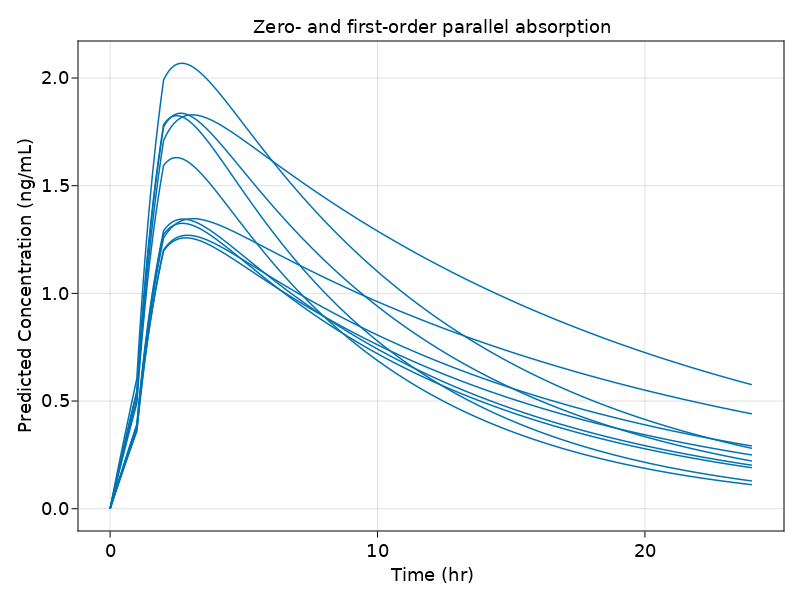## Absorption by Two Parallel First-Order Processes

This is similar to the example above, except that both parallel processes are first-order rather than one being zero-order. We will refer to the two processes as "immediate release" (IR) and "slow release" (SR); only the SR process is subject to a lag. The Pumas code is as follows:

two_parallel_foabs = @model begin

@param begin
tvcl  ∈ RealDomain(lower=0)
tvvc  ∈ RealDomain(lower=0)
tvka1 ∈ RealDomain(lower=0)
tvka2 ∈ RealDomain(lower=0)
tvlag ∈ RealDomain(lower=0)
tvbio ∈ RealDomain(lower=0)
Ω     ∈ PDiagDomain(6)
end

@random begin
η ~ MvNormal(Ω)
end

@covariates wt

@pre begin
CL  = tvcl*(wt/70)^0.75*exp(η)
Vc  = tvvc*(wt/70)*exp(η)
Ka1 = tvka1*exp(η)
Ka2 = tvka2*exp(η)
end

@dosecontrol begin
lags  = (SR = tvlag*exp(η),)
bioav = (IR = tvbio*exp(η), SR = (1 - tvbio)*exp(η))
end

@dynamics begin
IR'      = -Ka1*IR
SR'      = -Ka2*SR
Central' =  Ka1*IR + Ka2*SR - Central*CL/Vc
end

@observed begin
conc = @. Central/Vc
end

end

PumasModel
Parameters: tvcl, tvvc, tvka1, tvka2, tvlag, tvbio, Ω
Random effects: η
Covariates: wt
Dynamical variables: IR, SR, Central
Derived:
Observed: conc


Below are a set of parameter values for this model:

param = (
tvcl = 5, tvvc = 50, tvka1 = 0.8, tvka2 = 0.6, tvlag = 5, tvbio = 0.5,
Ω = Diagonal([0.04, 0.04, 0.36, 0.36, 0.04, 0.04]))

(tvcl = 5, tvvc = 50, tvka1 = 0.8, tvka2 = 0.6, tvlag = 5, tvbio = 0.5, Ω =
[0.04 0.0 … 0.0 0.0; 0.0 0.04 … 0.0 0.0; … ; 0.0 0.0 … 0.04 0.0; 0.0 0.0 …
0.0 0.04])


We can now use the data and model with parameters to simulate a profile with this type of absorption:

dose_fo1 = DosageRegimen(100, cmt = 1, time = 0)
dose_fo2 = DosageRegimen(100, cmt = 2, time = 0)
dose     = DosageRegimen(dose_fo1, dose_fo2)  # Actual dose is made up of 2 virtual doses

subj_with_covariates = map(1:10) do i
Subject(id = i,
events = dose,
covariates = choose_covariates(),
observations = (conc = nothing,))
end

sims = simobs(two_parallel_foabs, subj_with_covariates, param, obstimes = 0:.1:48)


And now, we plot the results:

sim_plot(two_parallel_foabs,
sims, observations =[:conc],
figure = (fontsize = 18, ),
axis = (xlabel = "Time (hr)",
ylabel = "Predicted Concentration (ng/mL)",
title = "Two Parallel first-order absorption"))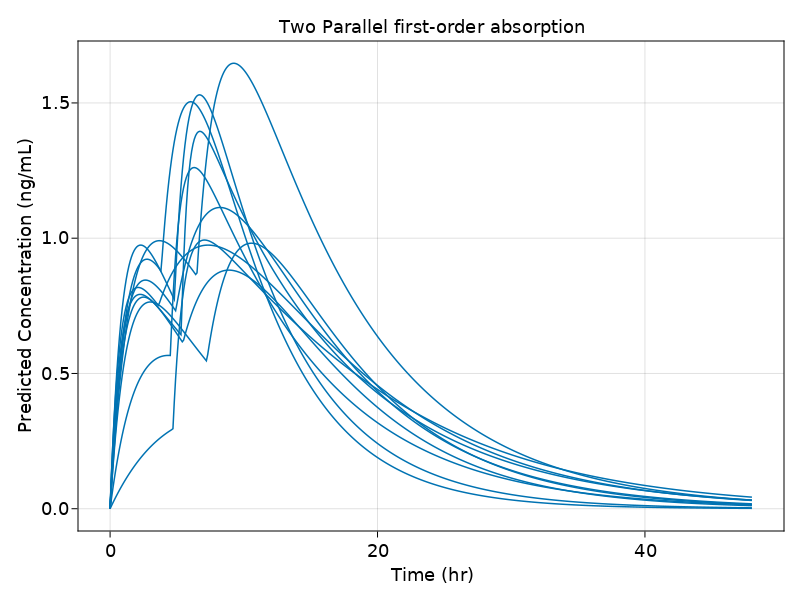## Weibull-Type Absorption

Yet other situations call for an absorption process that varies over time. One such model is this Weibull-type model, whereby the absorption is by a first-order process, but with a rate constant that is continuously changing over time according to a Weibull function (i.e., the CDF of a Weibull distribution). Here is the Pumas code for this model:

weibullabs = @model begin

@param begin
tvcl ∈ RealDomain(lower=0)
tvvc ∈ RealDomain(lower=0)
tvka ∈ RealDomain(lower=0)
tvγ  ∈ RealDomain(lower=0)
Ω    ∈ PDiagDomain(4)
end

@random begin
η ~ MvNormal(Ω)
end

@covariates wt

@pre begin
CL  = tvcl*(wt/70)^0.75*exp(η)
Vc  = tvvc*(wt/70)*exp(η)
Ka∞ = tvka*exp(η)           # Maximum Ka as t → ∞
γ   = tvγ*exp(η)            # Controls the steepness of the Ka curve
Kaᵗ = 1 - exp(-(Ka∞*t)^γ)      # Weibull function
end

@dynamics begin
Depot'   = -Kaᵗ*Depot
Central' =  Kaᵗ*Depot - (CL/Vc)*Central
end

@derived begin
conc = Central/Vc
end

end

PumasModel
Parameters: tvcl, tvvc, tvka, tvγ, Ω
Random effects: η
Covariates: wt
Dynamical variables: Depot, Central
Derived: conc
Observed: conc


Below are a set of parameter values for this model:

param = (
tvcl = 5, tvvc = 50, tvka = 0.4, tvγ = 4,
Ω = Diagonal([0.04, 0.04, 0.36, 0.04]))

(tvcl = 5, tvvc = 50, tvka = 0.4, tvγ = 4, Ω = [0.04 0.0 0.0 0.0; 0.0 0.04
0.0 0.0; 0.0 0.0 0.36 0.0; 0.0 0.0 0.0 0.04])


We can now use the data and model with parameters to simulate a Weibull-type absorption profile:

dose = DosageRegimen(100, cmt = 1, time = 0)

subj_with_covariates = map(1:10) do i
Subject(id = i,
events = dose,
covariates = choose_covariates(),
observations = (conc = nothing,))
end

sims = simobs(weibullabs, subj_with_covariates, param, obstimes = 0:.1:24)


And now, we plot the results:

sim_plot(two_parallel_foabs,
sims, observations =[:conc],
figure = (fontsize = 18, ),
axis = (xlabel = "Time (hr)",
ylabel = "Predicted Concentration (ng/mL)",
title = "Weibull absorption"))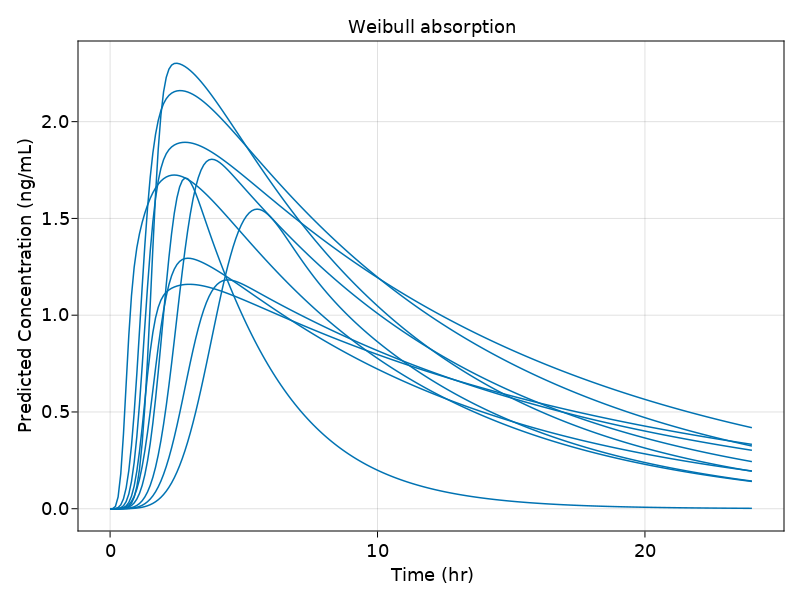## Erlang Absorption

The Erlang absorption model is based on the concept of transit compartments. The Erlang distribution is a special case of the Gamma distribution that arises as the sum of $k$ independent exponential distributions. This is exactly the distribution of the transit time taken by a particle passing through a sequence of $k$ compartments where the transition from one compartment to the next is governed by first-order processes with a common rate constant $K_{tr}$. In Pumas, this model can be written with the following code (we assume $N = 5$ transit compartments):

erlangabs = @model begin

@param begin
tvcl  ∈ RealDomain(lower=0)
tvvc  ∈ RealDomain(lower=0)
tvktr ∈ RealDomain(lower=0)
Ω     ∈ PSDDomain(3)
end

@random begin
η ~ MvNormal(Ω)
end

@covariates wt

@pre begin
CL  = tvcl*(wt/70)^0.75*exp(η)
Vc  = tvvc*(wt/70)*exp(η)
Ktr = tvktr*exp(η)
end

@dynamics begin
Depot'    = -Ktr*Depot
Transit1' = Ktr*Depot    - Ktr*Transit1
Transit2' = Ktr*Transit1 - Ktr*Transit2
Transit3' = Ktr*Transit2 - Ktr*Transit3
Transit4' = Ktr*Transit3 - Ktr*Transit4
Transit5' = Ktr*Transit4 - Ktr*Transit5
Central'  = Ktr*Transit5 - (CL/Vc)*Central
end

@observed begin
conc = @. Central/Vc
end

end

PumasModel
Parameters: tvcl, tvvc, tvktr, Ω
Random effects: η
Covariates: wt
Dynamical variables: Depot, Transit1, Transit2, Transit3, Transit4, Trans
it5, Central
Derived:
Observed: conc


Below are a set of parameter values for this model:

param = (
tvcl = 7, tvvc = 32, tvktr = 2.6,
Ω = Diagonal([0.09, 0.22, 0.10, 0.52]))

(tvcl = 7, tvvc = 32, tvktr = 2.6, Ω = [0.09 0.0 0.0 0.0; 0.0 0.22 0.0 0.0;
0.0 0.0 0.1 0.0; 0.0 0.0 0.0 0.52])


We can now use the data and model with parameters to simulate an Erlang absorption profile:

dose = DosageRegimen(100, cmt = 1, time = 0)

subj_with_covariates = map(1:10) do i
Subject(id = i,
events = dose,
covariates = choose_covariates(),
observations = (conc = nothing,))
end

sims = simobs(erlangabs, subj_with_covariates, param, obstimes = 0:1:24)


And now, we plot the results:

sim_plot(erlangabs,
sims, observations =[:conc],
figure = (fontsize = 18, ),
axis = (xlabel = "Time (hr)",
ylabel = "Predicted Concentration (ng/mL)",
title = "Erlang absorption"))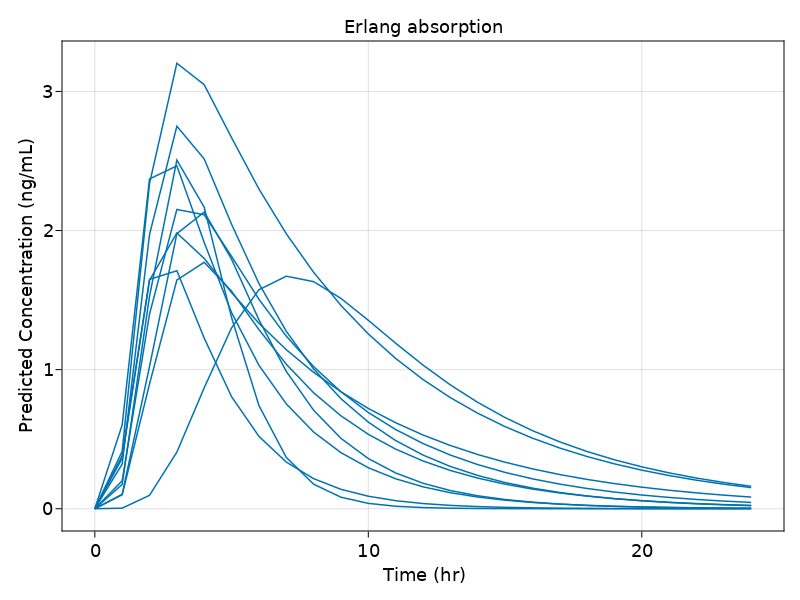## Conclusion

We have seen in this tutorial some examples of how to implement various absorption models that are commonly employed in pharmacokinetic modeling using Pumas. The powerful Pumas modeling language framework makes it relatively straightforward to implement these and other complex PK and PKPD models for modeling and simulation purposes.

This concludes our tutorial on basic absorption models with Pumas. Thanks for reading, and please be sure to check out our other tutorials as well.